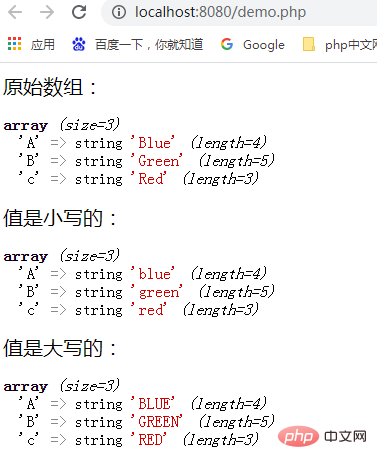# 如何将PHP数组的所有值更改为大写或小写

``````\$Color = array('A' => 'Blue', 'B' => 'Green', 'c' => 'Red');
``````

``````function array_change_value_case(\$input, \$ucase){
\$case = \$ucase;
\$narray = array();
if (!is_array(\$input)){
return \$narray;
}
foreach (\$input as \$key => \$value){
if (is_array(\$value)){
\$narray[\$key] = array_change_value_case(\$value, \$case);
continue;
}
\$narray[\$key] = (\$case == CASE_UPPER ? strtoupper(\$value) : strtolower(\$value));
}
return \$narray;
}
``````

PHP完整代码如下：

``````\$Color = array('A' => 'Blue', 'B' => 'Green', 'c' => 'Red');
echo '原始数组：';
var_dump(\$Color);
echo '值是小写的：';
\$myColor = array_change_value_case(\$Color,CASE_LOWER);
var_dump(\$myColor);
echo '值是大写的：';
\$myColor = array_change_value_case(\$Color,CASE_UPPER);
var_dump(\$myColor);
``````1、is_array()函数：检测变量是否是数组，其语法是“is_array(mixed \$var): bool”，如果var是 array，则返回true，否则返回false。

2、strtoupper()函数：用于把字符串转换为大写，该函数是二进制安全的，其返回值则是返回转换为大写的字符串。

3、strtolower()函数：用于把字符串转换为小写，该函数是二进制安全的，其返回值则是返回转换为小写的字符串。